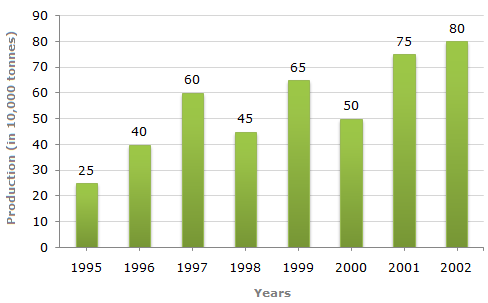# Data Interpretation - Bar Charts - Discussion

Study the bar chart and answer the question based on it.

Production of Fertilizers by a Company (in 1000 tonnes) Over the Years3.

What was the percentage increase in production of fertilizers in 2002 compared to that in 1995?

 [A]. 320% [B]. 300% [C]. 220% [D]. 200%

Explanation:

 Required percentage =(80 - 25) x 100% = 220%. 25

 Arjun said: (Dec 19, 2014) Here we divided by 25, in the question they ask increase in production. So we are going from lowest to top that's why we divided by 25. For another example if they ask decline from 2002 to 1995 then we divide the whole equation by 80 cause we are going top to lower. I hope its help you guys.

 Sangita said: (Sep 9, 2018) Thank you @Arjun.